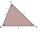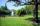Triangle ABC

Calculate the sides of triangle ABC with area 1404 cm2 and if a: b: c = 12:7:18

Result

a =  87.69 cm
b =  51.15 cm
c =  131.53 cm

Solution:Try calculation via our triangle calculator.

Leave us a comment of example and its solution (i.e. if it is still somewhat unclear...):Be the first to comment!To solve this example are needed these knowledge from mathematics:

Do you have a linear equation or system of equations and looking for its solution? Or do you have quadratic equation? Do you want to convert length units? See also our trigonometric triangle calculator.

Next similar examples:

1. Circles 2Calculate the area bounded by the circumscribed and inscribed circle in triangle with sides 12 cm, 14 cm, 18 cm.
2. DescribedCalculate perimeter of the circle described by a triangle with sides 478, 255, 352.
3. TrapezoidCalculate area of trapezoid ABCD with sides |AD|= 57 cm, |DC|=30 cm, |CB|=7 cm, |AB|=40 cm..
4. Heron backlawCalculate missing side in a triangle with sides 17 and 34 and area 275.
5. Triangular prismCalculate the surface of a triangular prism 10 cm high, the base of which is a triangle with sides 6 cm 8 cm and 8 cm
6. Sides of the triangleCalculate triangle sides where its area is S = 84 cm2 and a = x, b = x + 1, xc = x + 2
7. TriangleCalculate heights of the triangle ABC if sides of the triangle are a=75, b=84 and c=33.
8. Area and two anglesCalculate the size of all sides and internal angles of a triangle ABC, if it is given by area S = 501.9; and two internal angles α = 15°28' and β = 45°.
9. Cardboard boxWe want to make a cardboard box shaped quadrangular prism with rhombic base. Rhombus has a side of 5 cm and 8 cm one diagonal long. The height of the box to be 12 cm. The box will be open at the top. How many square centimeters cardboard we need, if we cal
10. Triangle SSSCalculate perimeter and area of ​​a triangle ABC, if a=40, b=36 and c=36.
11. TriangleTriangle KLM is given by plane coordinates of vertices: K[-12, -11] L[-15, -18] M[-13, -12]. Calculate its area and itsinterior angles.
12. PrismCalculate the volume of the rhombic prism. Base of prism is rhombus whose one diagonal is 47 cm and the edge of the base is 28 cm. The edge length of the base of the prism and height is 3:5.
13. Trapezoid - hard exampleBase of the trapezoid are: 24, 16 cm. Diagonal 22, 26 cm. Calculate its area and perimeter.
14. DiamondRhombus has side 17 cm and and one of diagonal 22 cm long. Calculate its area.
15. BoxCardboard box shaped quadrangular prism with a rhombic base. Rhombus has a side 5 cm and one diagonal 8 cm long and height of the box is 12 cm. The box will open at the top. How many cm2 of cardboard we need to cover overlap and joints that are 5% of ar
16. ParkIn the newly built park will be permanently placed a rotating sprayer irrigation of lawns. Determine the largest radius of the circle which can irrigate by sprayer P so not to spray park visitors on line AB. Distance AB = 55 m, AP = 36 m and BP = 28 m.
17. SailsWe known heights 220, 165 and 132 of sail. It has triangular shape. What is the surface of the sail?